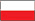The rules have been updated, read them now: Rules!

## Ben 10 Protector of Earth Compression Algorithm

Read or post about compression. And decompression. Or ask questions how to decompress your files.
theclub654
beginner
Posts: 27
Joined: Wed Oct 14, 2015 1:57 am
Has thanked: 3 times
Been thanked: 1 time

### Ben 10 Protector of Earth Compression Algorithm

If anyone is still interested in this game i found the compression algorithm in the game code i just dont know how to reverse it. This game doesnt use encryption by the way

ikskoks
Moderator
Posts: 813
Joined: Thu Jul 26, 2012 5:06 pm
Location: Poland, Łódź
Has thanked: 473 times
Been thanked: 216 times
Contact:

### Re: Ben 10 Protector of Earth Compression Algorithm

i found the compression algorithm in the game code
Oh, can you share with us?http://ikskoks.pl/
https://www.facebook.com/ikskokstheclub654
beginner
Posts: 27
Joined: Wed Oct 14, 2015 1:57 am
Has thanked: 3 times
Been thanked: 1 time

### Re: Ben 10 Protector of Earth Compression Algorithm

open up ida or ghidra or ida and go to address 1973E8 and that is the decompression routine if you set a break point on pcsx2 debugger at that address you can literally see the game decompressing the .wad file in your face

theclub654
beginner
Posts: 27
Joined: Wed Oct 14, 2015 1:57 am
Has thanked: 3 times
Been thanked: 1 time

### Re: Ben 10 Protector of Earth Compression Algorithm

If anyone is still interested in unpacking the compressed .wal file for ben 10 protector of earth this is the decompression routine as im not very good at reversing so maybe someone can give this a look and reverse it themselves i guess

Code: Select all

``````void FUN_001973e8(int param_1)

{
undefined *puVar1;
byte bVar2;
byte bVar3;
int iVar4;
uint uVar5;
bool bVar6;
int iVar7;
byte *pbVar8;
byte *pbVar9;
byte *pbVar10;
byte *pbVar11;
int iVar12;
undefined auStack80 ;
byte *pbStack64;
byte *pbStack60;
undefined auStack48 ;

pbVar9 = *(byte **)(param_1 + 0x44);
iVar12 = *(int *)(param_1 + 4) - (int)(pbVar9 + -0x10000);
iVar7 = iVar12 + -0x41;
if (*(int *)(param_1 + 0x3c) <= iVar12) {
iVar7 = *(int *)(param_1 + 0x3c);
}
pbVar11 = pbVar9 + iVar7;
pbVar10 = *(byte **)(param_1 + 0x40);
if (pbVar9 < pbVar11) {
bVar2 = *pbVar9;
while( true ) {
bVar3 = *pbVar9;
if ((char)bVar2 < '\0') {
pbVar8 = pbVar9 + 1;
pbVar9 = pbVar9 + 2;
iVar7 = ((*pbVar8 & 0xf) + 1) * 0x10000;
pbVar8 = pbVar10 + ((int)((uint)CONCAT11(bVar3,*pbVar8) << 0x10) >> 0x14) + -1;
do {
iVar7 = iVar7 + -0x10000;
bVar2 = *pbVar8;
pbVar8 = pbVar8 + 1;
*pbVar10 = bVar2;
pbVar10 = pbVar10 + 1;
} while (-1 < iVar7);
bVar6 = pbVar9 < pbVar11;
}
else {
iVar7 = (char)bVar2 * 0x1000000;
if ((char)bVar2 < '@') {
pbVar9 = pbVar9 + 1;
do {
iVar7 = iVar7 + -0x1000000;
bVar2 = *pbVar9;
pbVar9 = pbVar9 + 1;
*pbVar10 = bVar2;
pbVar10 = pbVar10 + 1;
} while (-1 < iVar7);
bVar6 = pbVar9 < pbVar11;
}
else {
bVar2 = pbVar9;
iVar7 = (bVar3 - 0x3e) * 0x1000000;
pbVar9 = pbVar9 + 2;
do {
iVar7 = iVar7 + -0x1000000;
*pbVar10 = bVar2;
pbVar10 = pbVar10 + 1;
} while (0 < iVar7 >> 0x18);
bVar6 = pbVar9 < pbVar11;
}
}
if (!bVar6) break;
bVar2 = *pbVar9;
}
}
puVar1 = auStack48 + 7;
uVar5 = (uint)puVar1 & 7;
*(ulong *)(puVar1 + -uVar5) =
*(ulong *)(puVar1 + -uVar5) & -1 << (uVar5 + 1) * 8 |
CONCAT44(pbVar10,pbVar9) >> (7 - uVar5) * 8;
auStack48._0_8_ = CONCAT44(pbVar10,pbVar9);
iVar7 = *(int *)(param_1 + 0x3c);
iVar4 = *(int *)(param_1 + 0x44);
pbStack64 = pbVar9;
pbStack60 = pbVar10;
auStack80._0_8_ = CONCAT44(pbVar10,pbVar9);
puVar1 = auStack80 + 7;
uVar5 = (uint)puVar1 & 7;
*(ulong *)(puVar1 + -uVar5) =
*(ulong *)(puVar1 + -uVar5) & -1 << (uVar5 + 1) * 8 | auStack80._0_8_ >> (7 - uVar5) * 8;
pbVar9 = pbVar9 + -iVar4;
iVar7 = iVar7 - (int)pbVar9;
iVar12 = iVar12 - (int)pbVar9;
*(byte **)(param_1 + 0x40) = pbVar10;
*(int *)(param_1 + 0x3c) = iVar7;
if (iVar7 == 0) {
pbVar9 = pbVar9 + iVar4;
}
else {
if (iVar12 != 0) {
FUN_001f927c(*(int *)(param_1 + 4) - iVar12,pbVar9 + iVar4,iVar12);
}
pbVar9 = (byte *)(*(int *)(param_1 + 4) - iVar12);
}
*(byte **)(param_1 + 0x44) = pbVar9;
return;
}

``````